# Branching process, age-dependent

(diff) ← Older revision | Latest revision (diff) | Newer revision → (diff)

A model of a branching process in which the lifetime of a particle is an arbitrary non-negative random variable, while the number of daughter particles depends on its age at the moment of transformation. In the single-type particle model each particle has a random duration of lifewith distribution functionAt the end of its life the particle is transformed intodaughter particles of age zero with a probability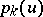if the transformation took place when the age attained by the original particle was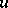. Let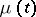be the number of particles at the moment of time. The generating function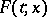of the probability distribution offor a process beginning with one particle of age zero satisfies the equation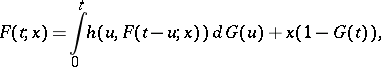(*)

where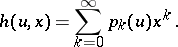Put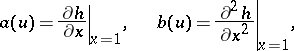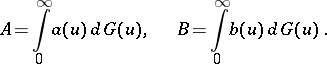An age-dependent branching process is said to be subcritical, critical or supercritical if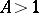,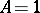and, or, respectively. The behaviour of the process as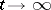substantially depends on its criticality. Subcritical and critical processes die out with probability one, i.e.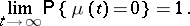The following results have been obtained for these processes : asymptotic formulas for the moments, necessary and sufficient conditions of extinction, conditions of existence and uniqueness of a solution of equation (*) and asymptotic formulas asfor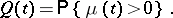The limit distributions have also been determined. In the critical case, as: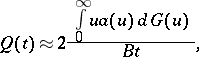Ifis independent of, the age-dependent branching process is a Bellman–Harris process. The model just described has been generalized to include processes with several types of particles, and also to processes for which a particle may generate new particles several times during its lifetime , .

How to Cite This Entry:
Branching process, age-dependent. Encyclopedia of Mathematics. URL: http://encyclopediaofmath.org/index.php?title=Branching_process,_age-dependent&oldid=13426
This article was adapted from an original article by V.P. Chistyakov (originator), which appeared in Encyclopedia of Mathematics - ISBN 1402006098. See original article